# Fractions + rational numbers - math problems

#### Number of problems found: 51

• FractionsSort fractions ? by its size. Result write as three serial numbers 1,2,3.
• CompareCompare fractions ?. Which fraction of the lower?
• FractionDetermine for what x fraction ?:
• Denominator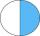Calculate the missing denominator x: ?
• One sixth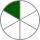How many sixths are two thirds?
• Determine the number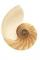Determine the number x that ?.
• Comparing and sortingArrange in descending order this fractions: 2/7, 7/10 & 1/2
• ExpressionIf it is true that ? is:
• Find the 11Find the quotient of 229.12 and 12.32
• FractionFraction ? write as fraction a/b, a, b is integers numerator/denominator.
• Ratio - proportion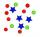Reduce the number 16 in proportion 3:2 5:4 11:8
• Lowest termsReduce to the lowest terms: 32/124
• Decimal to fractionWrite decimal number 8.638333333 as a fraction A/B in the basic form. Given decimal has infinite repeating figures.
• Package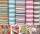The package was 23 meters of textile. The first day sold 12.3 meters. How many meters of textile remained in the package?
• What is one third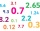What is 1/3 as a decimal? Give your answer rounded to 2 decimal places.
• DayWhat part of the day is 23 hours 22 minutes? Express as a decimal number.
• Company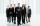After increasing the number of employees by 15% company has 253 employees. How many employees take?
• Rolls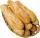Mom bought 13 rolls. Dad ate 3.5 rolls. How many rolls left when Peter yet put two at dinner?
• Infinite decimalImagine the infinite decimal number 0.99999999 .. ... ... ... That is a decimal and her endless serie of nines. Determine how much this number is less than the number 1. Thank you in advance.
• Mixed numbersRewrite mixed numbers so the fractions have the same denominator: 5 1/5 - 2 2/3

Do you have an interesting mathematical word problem that you can't solve it? Submit a math problem, and we can try to solve it.

We will send a solution to your e-mail address. Solved examples are also published here. Please enter the e-mail correctly and check whether you don't have a full mailbox.

Please do not submit problems from current active competitions such as Mathematical Olympiad, correspondence seminars etc...

Need help calculate sum, simplify or multiply fractions? Try our fraction calculator.